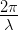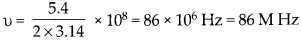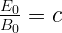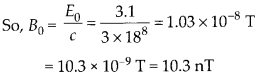Enlightened

# Question 11: NCERT Solutions for 12th Class Physics: Chapter 8-Electromagnetic Waves

• 0

Question 11: NCERT Solutions for 12th Class Physics: Chapter 8-Electromagnetic Waves

Suppose that the electric field part of an electromagnetic wave in vacuum is £ = [(3.1 N/C)] cos[(1.8 rad/m) y + (5.4 x 108 rad/s)f)] i.
(a) What is the direction of propagation?
(b) What is the wavelength λ?
(c) What is the frequency υ?
(d) What is the amplitude of the magnetic field part of the wave?
(e) Write an expression for the magnetic field part of the wave.

Share

1. Solution:
(a) Electromagnetic wave is propagating in -y direction.
(b) Propagation constant, k == 1.8(c) angular frequency, ω = 2πυ = 5.4 × 108(d) Amplitude of magnetic field pat,(e) Expression for magnetic field pat of waveCheck the complete chapter with solutions.

NCERT Solutions for 12th Class Physics: Chapter 8-Electromagnetic Waves

• 0